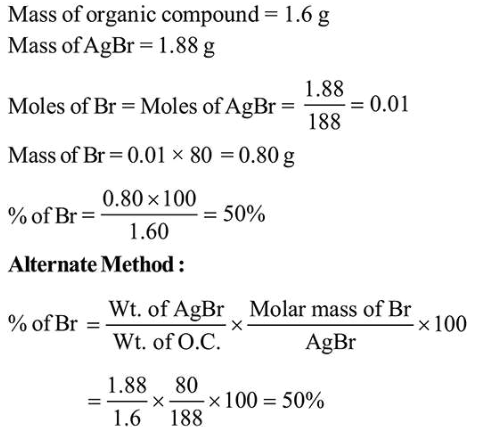# In an estimation of bromine by Carius method,Question:

In an estimation of bromine by Carius method, $1.6 \mathrm{~g}$ of an organic compound gave $1.88 \mathrm{~g}$ of $\mathrm{AgBr}$. The mass percentage of bromine in the compound is ______________ .(Atomic mass, $\mathrm{Ag}=108, \mathrm{Br}=80 \mathrm{~g} \mathrm{~mol}^{-1}$ )

Solution:

(50)